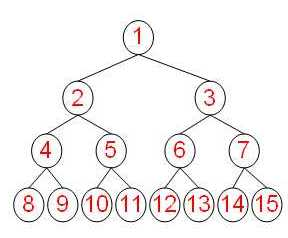Unique Studio Lab 第一期任务，在完成之后整理一下经验。PS:第一次接触C++，花了大半天速成C++，部分代码可能尚存在C语言的习惯。

## 定义和存储分析

• 每一个结点下方的数据都小于该结点
• 根结点拥有最高的优先级
• 具有O(log n)时间复杂度的插入元素性能，O(n)的初始化构造的时间复杂度。

## 基本方法与存储分析

• empty():判断队列是否为空。
• size(): 返回队列中数据的个数
• top(): 返回队列的头部数据
• push(const T&): 在队列尾部增加一个数据
• pop(): 队列头部的数据出队class Priority_queue {
public:

bool empty() const; //判断队列是否为空
size_t size() const; //返回队列中数据的个数
const T& top() const; //返回队列的头部数据
void push(const T& val); //在队列尾部增加一个数据
void pop(); //队列头部的数据出队
private:
vector<T> PriQueue; //存储类型vector
int currentsize = 0; //属性：当前队列大小
};



## 算法实现

### empty()

/* 判断队列是否为空,若为空，返回true */
bool Priority_queue::empty() const {
if (currentsize == 0)
return true;
else
return false;
}


### size()

/* 返回队列中数据的个数 */
size_t Priority_queue::size() const {
return currentsize;
}


### top()

/* 返回队列的头部数据 */
const T& Priority_queue::top() const {
return PriQueue;
}


### push(const T&)

/* 在队列尾部增加一个数据 */
void Priority_queue::push(const T& val) {
if (PriQueue.size() == 0)
PriQueue.resize(1);
if (currentsize == PriQueue.size() - 1) //重新分配空间
PriQueue.resize(PriQueue.size() * 2);
int k = ++currentsize; //k是新的空位置
for ( ; k > 1 && val > PriQueue[k / 2]; k /= 2)
PriQueue[k] = PriQueue[k / 2]; //上滤，把空位置上移
PriQueue[k] = val; //插入新值
}


### pop()

/* 队列头部的数据出队 */
void Priority_queue::pop() {
if (empty())
cout << "This priority queue is empty!" << endl;
else {
int i, child; //child是i的子结点序号
T temp = PriQueue[currentsize--]; //将最后一个位置的值储存
for (i = 1; i * 2 <= currentsize; i = child) {
child = i * 2;
if (child != currentsize && PriQueue[child] < PriQueue[child + 1]) //选择大的子结点
child++;
if (temp < PriQueue[child]) //下虑，把空位置下移
PriQueue[i] = PriQueue[child];
else
break;
}
PriQueue[i] = temp;
}
}


## 最终的头文件和实现

/* 说明，该优先队列以数据表示优先级，数据越大，表示优先级越高 */
#include <iostream>
#include <algorithm>
#include <vector>

using T = double; //数据类型，本次任务要求使用double
using std::vector; //存储类型使用vector
using std::cout;
using std::endl; //测试用输出

class Priority_queue {
public:

bool empty() const; //判断队列是否为空
size_t size() const; //返回队列中数据的个数
const T& top() const; //返回队列的头部数据
void push(const T& val); //在队列尾部增加一个数据
void pop(); //队列头部的数据出队
private:
vector<T> PriQueue; //存储类型vector
int currentsize = 0; //属性：当前队列大小
};


#include "priority_queue.h"

/* 判断队列是否为空,若为空，返回true */
bool Priority_queue::empty() const {
if (currentsize == 0)
return true;
else
return false;
}

/* 返回队列中数据的个数 */
size_t Priority_queue::size() const {
return currentsize;
}

/* 返回队列的头部数据 */
const T& Priority_queue::top() const {
return PriQueue;
}

/* 在队列尾部增加一个数据 */
void Priority_queue::push(const T& val) {
if (PriQueue.size() == 0)
PriQueue.resize(1);
if (currentsize == PriQueue.size() - 1) //重新分配空间
PriQueue.resize(PriQueue.size() * 2);
int k = ++currentsize; //k是新的空位置
for ( ; k > 1 && val > PriQueue[k / 2]; k /= 2)
PriQueue[k] = PriQueue[k / 2]; //上滤，把空位置上移
PriQueue[k] = val; //插入新值
}

/* 队列头部的数据出队 */
void Priority_queue::pop() {
if (empty())
cout << "This priority queue is empty!" << endl;
else {
int i, child; //child是i的子结点序号
T temp = PriQueue[currentsize--]; //将最后一个位置的值储存
for (i = 1; i * 2 <= currentsize; i = child) {
child = i * 2;
if (child != currentsize && PriQueue[child] < PriQueue[child + 1]) //选择大的子结点
child++;
if (temp < PriQueue[child]) //下虑，把空位置下移
PriQueue[i] = PriQueue[child];
else
break;
}
PriQueue[i] = temp;
}
}